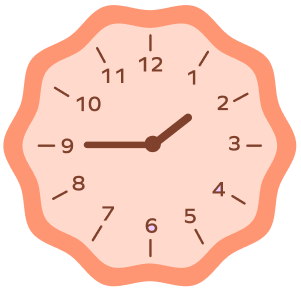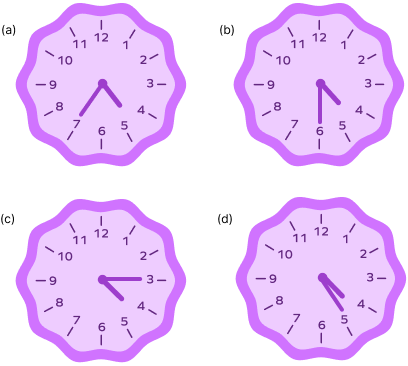# Quarter Hour in Math

Home » Math-Vocabluary » Quarter Hour in Math

## Quarter Hour: Introduction

Kamala went shopping with her mom. While driving home, she asked, “Mom, what’s the time?”

“A quarter past four,” her mom replied. Kamala had never heard of such a time before.

Cooking up something in her little head, she asked again, “Mom, will we reach home before Uncle Grandpa starts?”

“We’ll reach there by a quarter to five. Uncle Grandpa will start at quarter past five if I’m not wrong!” said her mom.

Will I reach home before Uncle Grandpa starts? she thought. Joe is still confused about the concept of “quarter-hour.” Let’s help her out!

In this article, we’ll see the definition of quarter-hours from a mathematical perspective and learn to tell quarter times.

## What Is One Quarter of an Hour?

One hour equals 60 minutes. If we divide one hour into four equal parts, each part is called a quarter of an hour and equals 15 minutes. Thus, one quarter of an hour means 14th of 60 minutes or simply 15 minutes.

## Quarters: Definition

The word “quarter” comes from the old French “quartier,” meaning a fourth. Therefore, “quarter” refers to one of the four equal divisions of something.

A quarter is 14th of a dollar and 14th of a yard. There are four quarters in a typical American football game and four quarts in a gallon.

## What Is a Quarter in Time?

A quarter in time refers to a fourth of a time block. For instance, a quarter of four hours is an hour. A quarter of eight hours is two hours.

## What Is a Quarter of an Hour?

A quarter hour refers to a fourth of an hour. If we divide an hour into four equal time patches, each would equal a quarter-hour.

## How Long Is a Quarter of an Hour?

One hour is 60 minutes. So, a quarter hour is 14th of 60, i.e., 15 minutes. Therefore, a quarter hour refers to fifteen minutes.

## Analog Clocks and Digital Clocks

Digital clocks show us the exact time in hours and minutes. However, in analog clocks, we have to understand the time by looking at the positions of the hour and minute hands. In this section, we’ll try to convert the time in analog clocks to digital form.

To read analog clocks and convert the time to digital form, you may follow the below steps:

1. There are at least two components in analog time—the hour component and minute component
2. Note the position of the hour hand and the minute hand.
3. The hour component is the position of the hour hand in the clock.
4. One hour is 60 minutes. So, the distance between two consecutive notches of the clock is 6012 = 5 minutes. So, to get the minute component, you must multiply the minute hand’s position by 5.
5. Finally, write the digital time as “hour component: minute component.”

Example 1: Convert the following time into digital form.

The hour hand is at two (hasn’t reached or crossed 3), and the minute hand is at 6. The hour component is 2, and the minute component is 6 x 5 = 30. Therefore, the digital version of this time is 2:30.

### Quarter-hour Fractions in Analog Clocks

We can divide an analog clock into four equal parts. Each part represents a quarter time. Concerning its minute hand, each part represents a quarter hour.

Example 2: The clock below shows the passing of a quarter-hour from 12.

## Telling Time: Quarter Hour

We can tell quarter times in two ways. Let’s see each of them.

### Quarter Past

“Quarter past” refers to a quarter-hour past (after) the given time. For instance, quarter past eight refers to 15 minutes after 8 or 8:15.

Observe that in a quarter past the hour, the hour hand will be slightly ahead of the number.

### Quarter To

“Quarter to” refers to a quarter-hour to (before) the given time. For instance, quarter to eleven refers to 15 minutes to 11 or 10:45.

Observe that in quarter to an hour the hour hand will be slightly behind the number.

I’m a clock,

My hands race.

Minute is fast and fiery,

But the Hour is slow and steady.

Quarter to?

Minute, fifteen minutes to win!

And quarter past?

Minute, come back!

## Fun Facts!

• Quarters not only exist for perfect times. For instance, a quarter past 2:41 p.m. is 2:56 p.m.
• In Hands Across America, a fundraising event held on 25th May 1986 in the US, around 6 million people held their hands to form a human chain for a quarter-hour.
• The clock at Lambeau Field is always a quarter-hour early. It is to honor Lombardi, the legendary coach who believed one is late if they aren’t a quarter-hour early.
• At 9 a.m., a group of bagpipers wakes the British Monarch by playing bagpipes for a quarter of an hour.

## Conclusion

A quarter hour is a unit of time that is equal to a quarter (one-fourth) of an hour or 60 minutes.

Knowing the concept of a quarter hour thus helps in telling time, reading time and calculating time.

## Solved Examples

1. What is the time shown in the clock below?

Solution:

In the figure, the hour hand of the clock is at 1. So, the hour component of the digital time is also 1.

Also, on the clock, the minute hand is at 3. So, the minute component of the digital time is 3 x 5 = 15.

So, the time is 3:15 or 15 minutes past 1. We can write it as a quarter past one.

2. Write the digital time of ‘quarter to five’.

Solution:

15 minutes to 5 is 4:45.

3. What is two quarters of an hour in minutes?

Solution:

A quarter-hour is 15 minutes. So, two quarters will be 30 minutes or half an hour.

4. Lombardi believed that one is late if they aren’t a quarter-hour early. So, by what time must a player reach the field if they have to arrive at 6:20 AM for a drill?

Solution:

Lombardi believed that one is late if they aren’t a quarter-hour early.

So, to avoid being late, the player should be a quarter-hour or 15 minutes early before the scheduled time.

15 minutes before 6:20 a.m. is 6:05 a.m.

5. It’s quarter past 4. Aaron is heading back home with his mother to see his grandpa who will leave by quarter past 5. Aaron was supposed to reach home by quarter to 5, but while driving back home, Aaron’s mom encounters a traffic jam that lasts a quarter of an hour. Will Aaron be able to reach his house to meet his grandpa on time?

Solution:

The current time is quarter past four or 4:15 p.m.

Aaron was supposed to reach home by quarter to 5 or 4:45 p.m.

Now, if Aaron’s mom encounters a traffic jam that lasts a quarter of an hour, they’ll reach home 15 minutes. That is, they will now reach home by 5 p.m.

And since Aaron’s grandpa leaves at quarter past 5, or 5:15 p.m., Aaron will be home in time to see him off.

## Practice Problems

1

### The clock given below shows:Quarter past 2
Quarter to 2
Quarter past 1
Quarter to 1
CorrectIncorrect
Correct answer is: Quarter to 2
The time shown on the clock is 1:45, which is a quarter to two.
2

### At 9 a.m., a group of bagpipers wakes the British monarch by playing pipers for a quarter of an hour. Till what time will they continue playing?

Quarter to 9
9:15 a.m.
Quarter to till 9
8:45 a.m.
CorrectIncorrect
The pipers, starting from 9, will play the pipes for 15 minutes till 9:15 a.m.
3

### Which of the following time stamps is the same as “half past ten”?

10:15
10:00
10:45
10:30
CorrectIncorrect
If a quarter-hour is 15 minutes, two quarters (or half) is 30 minutes, then half past 10 means 10:30.
4

### The hour hand of a clock is at 10. In which of the following instances is the time lapsed equal to a quarter-hour?

The minute hand moving from 2 to 5
The minute hand moving from 7 to 10
The minute hand moving from 11 to 2
All of the above
CorrectIncorrect
Correct answer is: All of the above
From 2 to 5, 7 to 10, and 11 to 2, the minute hand is moving 3 notches $= 3 \times 5 = 15$ minutes. So, all the given instances represent a quarter-hour time lapse.
5

### Which of the following clocks show “quarter past four”?a
b
c
d
CorrectIncorrect
Quarter past four is 4:15, and clock (c) shows the same.

No. A quarter-hour is 15 minutes.

30 minutes will be two quarters or half an hour.

A quarter-hour is 1/4th or 25% of an hour.

No. A quarter past the hour is the first quarter. Half past the hour is the second, three quarters past the hour is the third, and a quarter to the hour is the fourth quarter.

There are four quarter-hours in an hour. And there are 24 hours in a day.

So, there are $24 \times 4 = 96$ quarter-hours in a day.

There are 15 minutes in a quarter-hour.

Every minute has 60 seconds.

So $15 \times 60 = 900$ seconds in a quarter-hour.